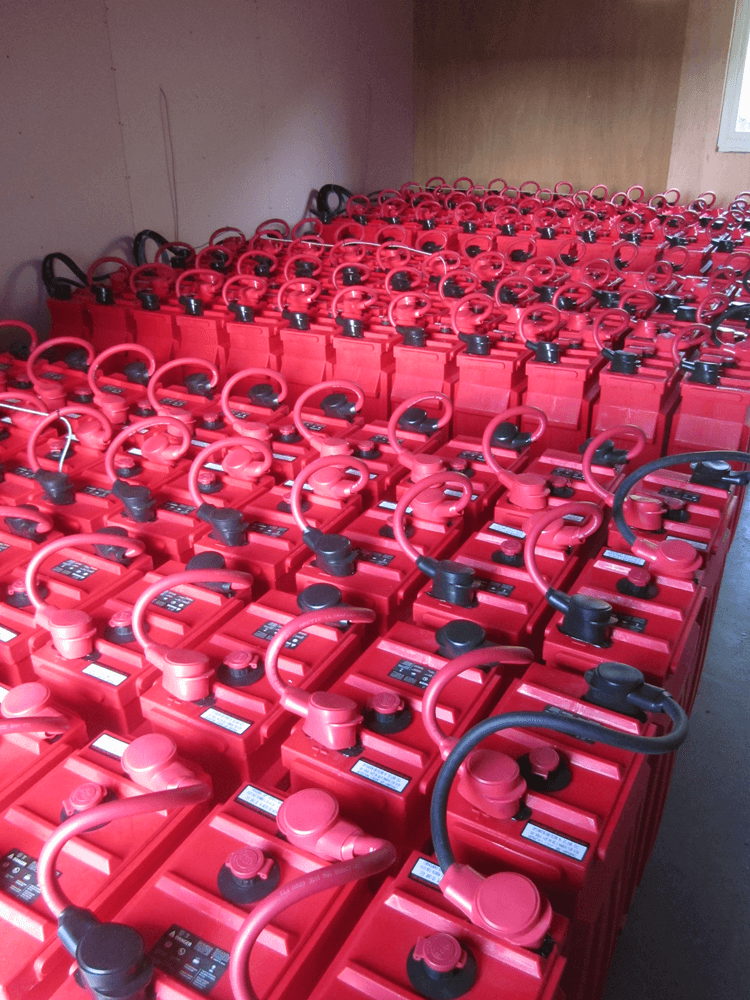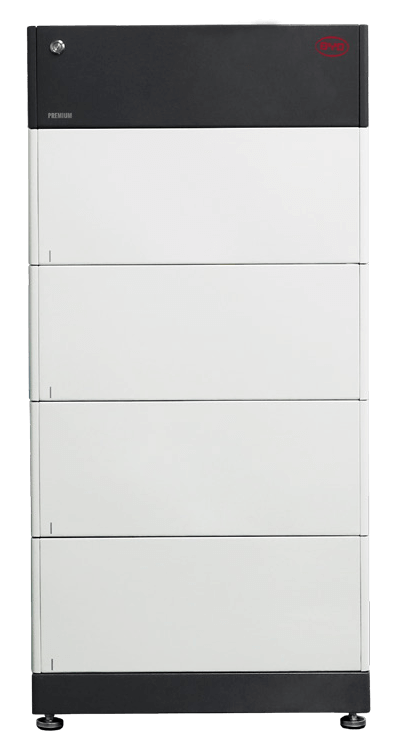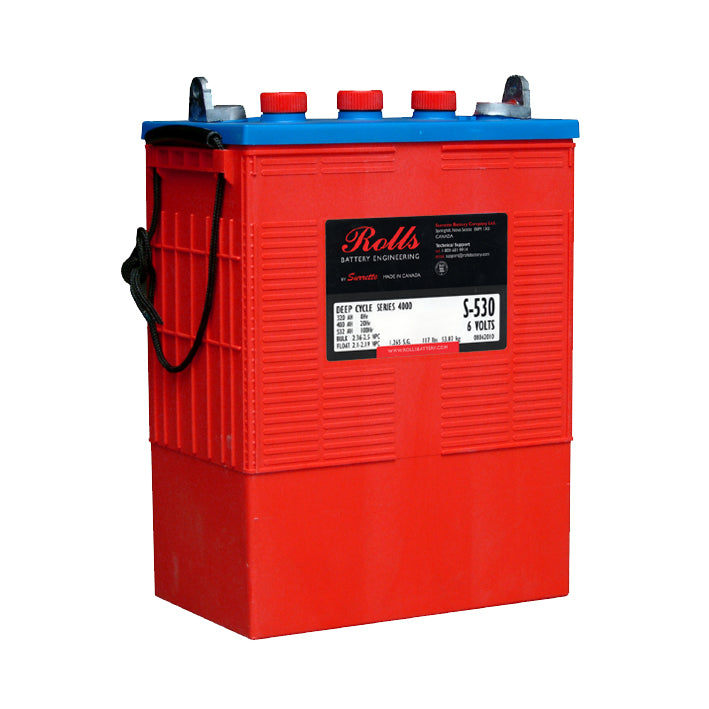# Battery Capacity

07 Jun 23

### ContentsThis is how much electrical energy the battery can deliver and is measured in ampere hours (Ah) or kilowatt hours (kWh).

Voltage (V) x Amp hours (Ah) = Watt Hours (Wh) ; 1000 Wh = 1 kWh

## Nominal Capacity

This is provided by the manufacturer and is a measure of how much energy the battery can deliver from fully charged, under certain conditions.

Battery capacity is normally described in Amp-Hour at a particular discharge current (C-rate), for example: 500Ah at C100 rate.

## C-Rate

The C-rate is a measure of the rate at which a battery is discharged compared to its nominal capacity. A 1C rate (or a rate of C1) means that the discharge current will completely discharge the battery in 1 hour; a rate of C20 equates to the current that will completely discharge the battery in 20 hours.

For example:

• For a battery with a capacity of 500Ah at the C100 rate this equates to a discharge current of 5 Amps.
• For a battery rated at 500Ah at the C1 rate, this means a discharge current of 500A.

The C10 rate is usually the best rate to use when considering batteries for a solar PV system.

When comparing battery options, it is important that capacities are compared using the same C-rate for the quoted capacity.

The C-rate can have a significant effect on battery capacity, particularly with lead-acid batteries. In general, the quicker a battery is discharged, the smaller its capacity.

If discharged at a higher rate (by a higher current) then battery capacity is reduced considerably. The maximum charge/discharge current should be less than 10% of battery Ah capacity.

## Depth of Discharge

Depth of discharge (DOD) is a measure of how much energy is taken out of the battery during a discharge cycle expressed as a percentage of battery capacity.

A depth of discharge of around 80% is known as a “deep cycle”.

Especially for lead acid batteries DOD can have a significant impact on battery lifetime. Different batteries are described as having a lifetime of a certain number of cycles to a certain DOD The higher the DOD, the shorter the lifetime. Consequently for long life it is important to choose appropriate batteries and limit the routine depth of discharge.

Deep cycle lead-acid batteries typically can be cycled regularly to ~ 50% DOD.

Most lithium-ion batteries can be discharged to around 80% of nominal capacity without significant effect on lifetime.

## Useable Capacity

Since depth of discharge should be limited, it is better to describe the usable capacity of the battery.

For example a battery with a 500Ahr capacity and permitted DOD of 60%; the usable capacity is 500 x 0.6 = 300Ah

If the battery voltage is 48V this equates to 48 x 300 = 14.4kWh

## Required Capacity

The capacity of the battery bank needed depends upon:

• amount of storage required
• types of charging source
• maximum charge & discharge rates
• temperature of operation### Products#### Lithium Batteries

View collection